# Weekday Abbreviations in Excel & Google Sheets

This tutorial will demonstrate how to use Weekday Abbreviations in Excel and Google Sheets.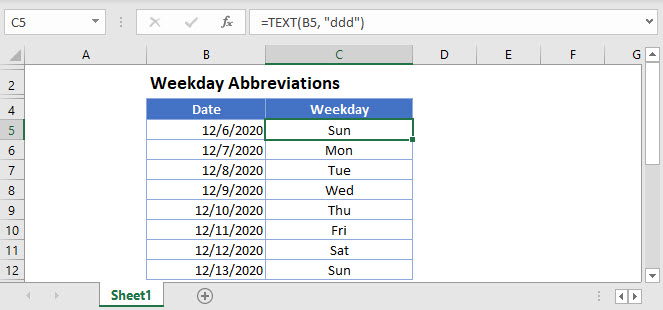## TEXT, CHOOSE and WEEKDAY Functions

To get the weekday abbreviation from a date, we can use the TEXT function, or we can use the CHOOSE and WEEKDAY functions.

### TEXT Function

We can use the TEXT function to obtain the weekday abbreviation.

``=TEXT(B3, "ddd")``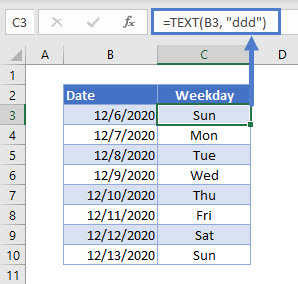In Excel, dates are stored as serial numbers, where each number represents an unique date. The TEXT Function converts a number into a specified text format. Here we specify the format “ddd” to get the day name abbreviation of a date.

### CHOOSE and WEEKDAY functions

Alternatively, we can use the CHOOSE and WEEKDAY functions to create a custom weekday abbreviation.

``=CHOOSE(WEEKDAY(B3),"S","M","T","W","T","F","S")``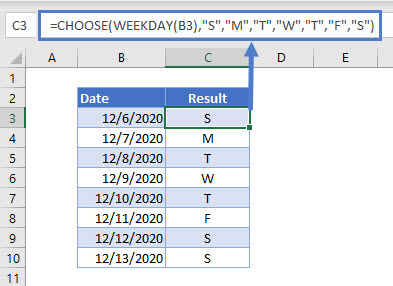### WEEKDAY function

First, we can get the weekday from cell B3

``=WEEKDAY(B3)``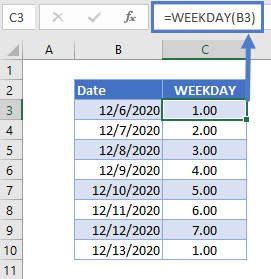### CHOOSE function

Next, we can use the CHOOSE function to choose the position of the abbreviation from the listed options, based on the value returned by the weekday function.

``=CHOOSE(WEEKDAY(C3),"S","M","T","W","T","F","S")``Joining these 2 formulas together gives us our result.

``=CHOOSE(WEEKDAY(B3),"S","M","T","W","T","F","S")``## Weekday Abbreviations in Google Sheets

All the above examples work the same way in Google Sheets.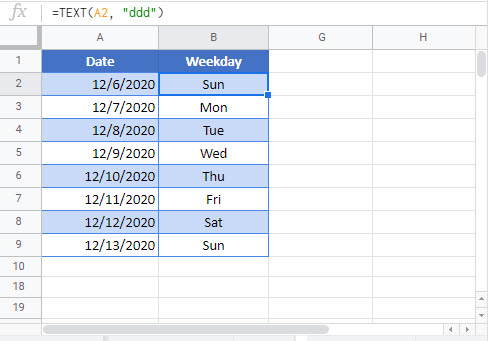### Excel Practice Worksheet

Practice Excel functions and formulas with our 100% free practice worksheets!